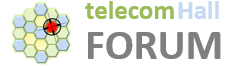Hello,

In our tool, we can configure serving cell and neighbours. And the config has transmit powers for the serving and neighbours.
Lets say one serving and one neighbours are configured.
Now in our code, i can see they are constructing the two different types of subframes (based on cyclic prefix, pci etc)

In the following code:
void Populate(const CSubFrame& A, const CSubFrame& B)
{
Clear();

``````	const bool bMbsfnA	= A.GetNumSymbolsNonMBSFN() != ALL_UNICAST;
const char markerA	= ( bMbsfnA ) ? 'R' : 'r';	// Only want to consider columns where the victim subframe 'A' contains this reference symbol

double dBoxesA(0), dBoxesB_r(0), dBoxesB_t(0), dBoxesB_R(0), dBoxesB_T(0);
for (size_t iX = 0; iX < NUMBOXES_X; iX++)	// Loop columns
{
bool bColumnContainsMarkerA(false);
for (size_t iY = 0; iY < NUMBOXES_Y && !bColumnContainsMarkerA; iY++)	// Loop rows in column
{
bColumnContainsMarkerA = ( A.GetBox(iY, iX) == markerA );
}
if ( !bColumnContainsMarkerA ) continue;	// Skip column if it doesn't contain markerA

for (size_t iY = 0; iY < NUMBOXES_Y; iY++)	// Loop rows in column
{
switch( B.GetBox(iY, iX) )	// Read interferer 'box' and update interferer box count
{
case 'r':	dBoxesB_r += 1.0;	break;
case 't':	dBoxesB_t += 1.0;	break;
case 'R':	dBoxesB_R += 1.0;	break;
case 'T':	dBoxesB_T += 1.0;	break;
}
}
dBoxesA += NUMBOXES_Y;	// Update victim box count.  (Every box in the column is a victim).
}
ASSERT_ONCE(dBoxesA > 0);	// If this blows we must must have no reference signals in the subframe !!!
if ( dBoxesA > 0 )
{
// Every useful RE consists of 11.2 boxes, regardless of subframe config, so in order to calculate
// the number of interfering REs (without CP) per victim RE (without CP) we can just take a ratio of the box counts

const double dFactor = 1.0 / dBoxesA;
m_dNumREs_t	= dBoxesB_t * dFactor;	// Mean number of interfering 't' REs per victim RE
m_dNumREs_r	= dBoxesB_r * dFactor;	// Mean number of interfering 'r' REs per victim RE
m_dNumREs_T	= dBoxesB_T * dFactor;	// Mean number of interfering 'T' REs per victim RE
m_dNumREs_R	= dBoxesB_R * dFactor;	// Mean number of interfering 'R' REs per victim RE
}
}
``````

we pass the serving cell frame and neighbour frame.
As you can see it skips the columns that does not have reference symbols.

As you can see each it counts the no of traffic and reference signal for the neighbour cell.

And divides that with the total number of blocks on the serving cell. to get the mean number of reference IEs per victim RE.

I feel something is not right. May be Im new to phy and i might have not understood the concept of why this is being done.

Could some physical developers help me with their inputs.

thanks
pdk